This note will de­rive the La­grangian mul­ti­pli­ers for an ex­am­ple prob­lem. Only cal­cu­lus will be used. The ex­am­ple prob­lem will be to find a sta­tion­ary point of a func­tionof four vari­ables if there are two con­straints. Dif­fer­ent num­bers of vari­ables and con­straints would work out in sim­i­lar ways as this ex­am­ple.

The four vari­ables that ex­am­ple func­tionde­pends on will be de­noted by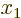,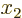,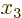, and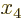. The two con­straints will be taken to be equa­tions of the form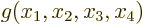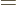0 and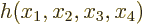0, for suit­able func­tions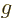and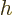. Con­straints can al­ways be brought in such a form by tak­ing every­thing in the con­straint’s equa­tion to the left-hand side of the equals sign.

So the ex­am­ple prob­lem is: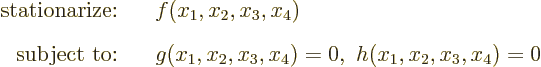Sta­tion­ar­ize means to find lo­ca­tions where the func­tion has a min­i­mum or a max­i­mum, or any other point where it does not change un­der small changes of the vari­ables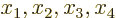as long as these sat­isfy the con­straints.

The first thing to note is that rather than con­sid­er­ingto be a func­tion of, you can con­sider it in­stead to be to be a func­tion ofandand only two ad­di­tional vari­ables from, sayand: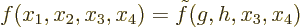The rea­son you can do that is that you should in prin­ci­ple be able to re­con­struct the two miss­ing vari­ablesandgiven,,, and.

As a re­sult, any small change in the func­tion, re­gard­less of con­straints, can be writ­ten us­ing the ex­pres­sion for a to­tal dif­fer­en­tial as: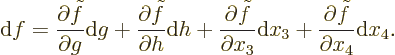At the de­sired sta­tion­ary point, ac­cept­able changes in vari­ables are those that keepandcon­stant at zero; they have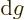0 and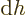0. So forto be sta­tion­ary un­der all ac­cept­able changes of vari­ables, you must have that the fi­nal two terms are zero for any changes in vari­ables. This means that the par­tial de­riv­a­tives in the fi­nal two terms must be zero since the changes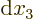and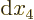can be ar­bi­trary.

For changes in vari­ables that do go out of bounds, the change inwill not be zero; that change will be given by the first two terms in the right-hand side. So, the er­ro­neous changes indue to go­ing out of bounds are these first two terms, and if we sub­tract them, we get zero net change for any ar­bi­trary change in vari­ables: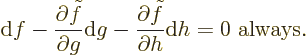In other words, if we pe­nal­ize the change infor go­ing out of bounds by amountsandat the rate above, any change in vari­ables will pro­duce a pe­nal­ized change of zero, whether it stays within bounds or not.

The two de­riv­a­tives at the sta­tion­ary point in the ex­pres­sion above are the La­grangian mul­ti­pli­ers or penalty fac­tors, call them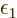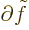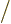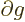and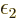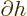. In those terms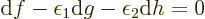for what­ever is the change in the vari­ables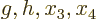, and that means for what­ever is the change in the orig­i­nal vari­ables. There­fore, the change in the pe­nal­ized func­tion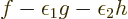is zero what­ever is the change in the vari­ables.

In prac­ti­cal ap­pli­ca­tion, ex­plic­itly com­put­ing the La­grangian mul­ti­pli­ersandas the de­riv­a­tives of func­tion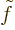is not needed. You get four equa­tions by putting the de­riv­a­tives of the pe­nal­izedwith re­spect tothroughequal to zero, and the two con­straints pro­vide two more equa­tions. Six equa­tions is enough to find the six un­knownsthrough,and.